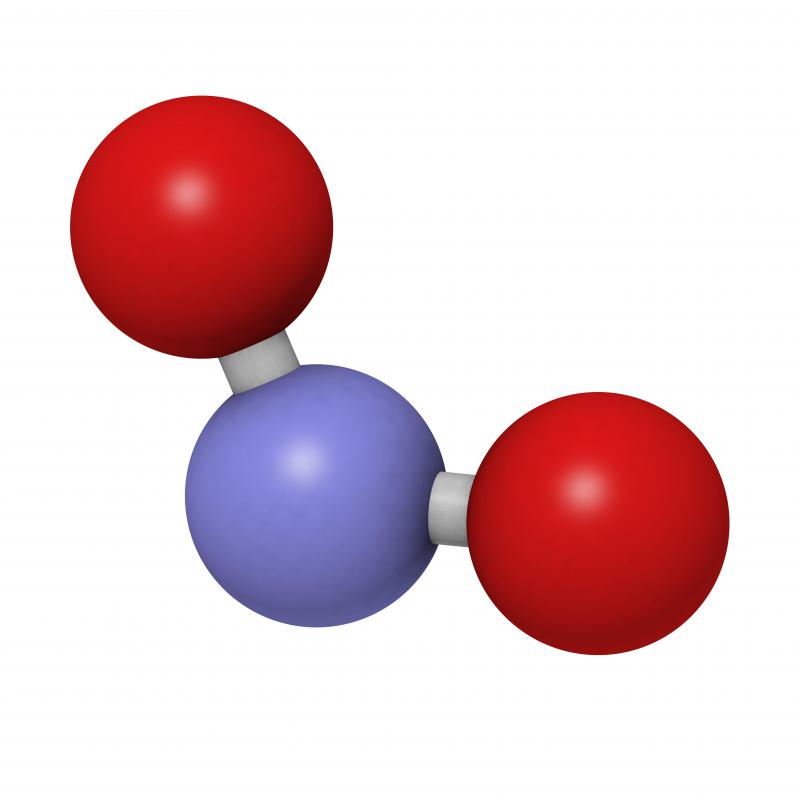# What is the Atomic Mass Unit?

Jason C. Chavis

The atomic mass unit is the system of measurement designed to identify each individual unit of mass in atoms and molecules. Also known as a dalton, the atomic mass unit is a universally-applied measurement based on 1/12 the total mass of a single carbon-12 atom. This means that a carbon-12 atom has the atomic mass of 12 daltons. The designation for a standard atomic mass unit is u or Da. Atomic mass units are used as the system of measurement in every science, except for those involving biology and biochemistry, which use the dalton designation.A single water molecule is made up of two hydrogen atoms and one atom of oxygen.

One convenient aspect of atomic mass units is that, while based on carbon mass, a single unit is also equal to one hydrogen atom. This is because the combined mass of a single proton and neutron, the composition of a hydrogen atom, is equal to the measurement. Electrons, being only 1/1836 the mass of a proton, are essentially negligible to the overall mass of an atom.

One of the most problematic aspects to using the atomic unit of mass to define atoms is that it does not account for the energy that binds together an atom's nucleus. Unfortunately, this is not a fixed mass due to the differences between each different types of atom. As more protons, neutrons and electrons are added to an atom to create a new element, the mass of this binding energy changes. This means that the measurement can be said to be a rough approximation rather than an exact constant.

One of the main uses for the atomic mass unit involves its relationship with moles. A mole is the complete physical quantity of a single unit of a substance. For example, a single water molecule, comprised of two hydrogen atoms and a single oxygen atom, is a mole of water. This means that it has the atomic mass of all three atoms.

The establishment of the atomic mass unit was first started by a chemist name John Dalton in the early 1800s. He used a single hydrogen atom as the platform for the measurement. However, this was altered by Francis Aston with his invention of the mass spectrometer in the late 1800s. Aston defined an atomic mass unit as being 1/16 the mass of a single oxygen-16 atom. It wasn't until 1961 that the International Union of Pure and Applied Chemistry defined the modern applications of the measurement and linked it to carbon-12.

## You might also LikeValleyFiah

The atomic mass of an element is actually proportional average of all the different naturally occurring isotopes of an element. These isotopes all have different atomic masses, so when an atomic mass is given for an element, it is a proportional average of the different isotopes.

As for determining the mass of a number of moles of a compound, there are a couple of easy steps to follow. Your example methane has an atomic mass of 16.06 g/mol. This tells you that for every mole of methane there are 16.06 grams of methane. You can simply multiply this number by the number of moles of methane you need to determine how many grams of methane you need. In this case, 0.63 moles of methane is equal to 10.12grams of methane when rounded to four significant figures.

Alchemy

Why do some people refer to the atomic mass of an element as the atomic mass average? Also how would I calculate the molecular weight of a fraction of a mole of a chemical compound, say 0.63 moles of methane?

GlassAxe

A mole of a substance is a bit more complicated than explained. A mole of water is much more than one molecule of water. It is actually 6.022x10^23 molecules of water. A mole is actually a proportionality constant scientifically defined by Avogadro's constant (6.022x10^23). A mole is most useful when dealing with chemical reactions because it keeps chemicals in proportion with their chemical equations while eliminating ridiculously large or small numbers. It is easy to convert moles to grams when dealing in mole measurements.

A mole can be used to measure anything, but one mole of a substance is the equivalent of the atomic mass number of that substance in grams. For example, one mole of oxygen is 15.999 grams, one mole of hydrogen has a mass of 1.01 grams, one mole of carbon has a mass of 12.02 grams, and so on.